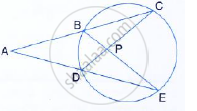Share

In the Given Figure, Ac = Ae Show That: (I) Cp = Ep (Ii) Bp = Dp - ICSE Class 10 - Mathematics

ConceptTangent Properties - If a Line Touches a Circle and from the Point of Contact, a Chord is Drawn, the Angles Between the Tangent and the Chord Are Respectively Equal to the Angles in the Corresponding Alternate Segments

Question

In the given figure, AC = AE Show that:
(i) CP = EP
(ii) BP = DPSolution

In ΔADC and ΔABE ,
∠ACD = ∠AEB (angles in the same segment)
AC = AE (Given)
∠A = ∠A(common)
∴ Δ ADC ≅  Δ ABE (ASA postulate)
⇒ AB = AD
But AC = AE
∴ AC – AB = AE – AD
⇒  BC = DE
In Δ BPC and ΔDPE
∠C =  ∠E (angles in the same segment)
BC = DE
∠CBP = ∠CDE (angles in the same segment)
∴ ΔBPC ≅  ΔDPE (ASA Postulate)
⇒ BP = DP and CP = PE (cpct)

Is there an error in this question or solution?

Video TutorialsVIEW ALL 

Solution In the Given Figure, Ac = Ae Show That: (I) Cp = Ep (Ii) Bp = Dp Concept: Tangent Properties - If a Line Touches a Circle and from the Point of Contact, a Chord is Drawn, the Angles Between the Tangent and the Chord Are Respectively Equal to the Angles in the Corresponding Alternate Segments.
S